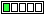All about flooble | fun stuff | Get a free chatterbox | Free JavaScript | Avatarsperplexus dot infoFibo's quickie (Posted on 2014-09-03)You have 20 seconds (starting at the end of reading the puzzle)
to validate or to disprove the following formula of calculating
the area of a triangle:

S=1/3* ((2AB/C)+(2AC/B)+(2BC/A)).

where the sides A,B & C are represented by three different Fibonacci numbers.Comments: ( Back to comment list | You must be logged in to post comments.)Pie are squared| Comment 2 of 4 |20 seconds of dimensional analysis are more than sufficient to disprove the above formula.

Areas must have units equal to length squared, but this formula has units equal to length, not length squared.

For instance, if A and B and C are in inches, the S is also in inches, so it cannot be an area.  It might be some sort of formula for perimeter, or semi-perimeter, or harmonic mean of the sides, or some other metric that has a dimension of length, but definitely not area.

It took longer to write this down than to it took to figure out.

And, by the way, that holds for any ABC, not just fibonacci numbers.  So I have proved (or disproved) more than requested.  Extra credit, Ady?

 Posted by Steve Herman on 2014-09-03 13:18:51Please log in:

 Search: Search body:
Forums (0)